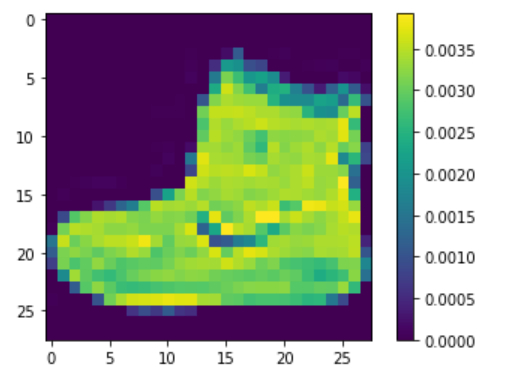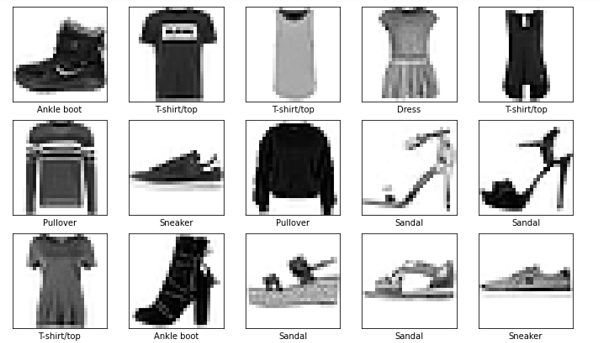# How can TensorFlow be used to preprocess Fashion MNIST data in Python?

Tensorflow is a machine learning framework that is provided by Google. It is an open−source framework used in conjunction with Python to implement algorithms, deep learning applications and much more. It is used in research and for production purposes.

The ‘tensorflow’ package can be installed on Windows using the below line of code −

pip install tensorflow

Tensor is a data structure used in TensorFlow. It helps connect edges in a flow diagram. This flow diagram is known as the ‘Data flow graph’. Tensors are nothing but multidimensional array or a list.

The ‘Fashion MNIST’ dataset contains images of clothing of different kinds. It contains grayscale images of more than 70 thousand clothes that belong to 10 different categories. These images are of low resolution (28 x 28 pixels). We are using the Google Colaboratory to run the below code. Google

Colab or Colaboratory helps run Python code over the browser and requires zero configuration and free access to GPUs (Graphical Processing Units). Colaboratory has been built on top of Jupyter Notebook.

Following is the code snippet −

## Example

plt.figure()
plt.imshow(train_images)
plt.colorbar()
plt.grid(False)
plt.show()

train_images = train_images / 255.0

test_images = test_images / 255.0

plt.figure(figsize=(12,12))
for i in range(15):
plt.subplot(5,5,i+1)
plt.xticks([])
plt.yticks([])
plt.grid(False)
plt.imshow(train_images[i], cmap=plt.cm.binary)
plt.xlabel(class_names[train_labels[i]])
plt.show()

## Output## Explanation

• Before the network is trained, the input dataset needs to be processed.

• Once the images are inspected and displayed on the console, it can be determined that the pixel values fall within the range of 0 to 255.

• These pixel values are first scaled to fall within the range of 0 to 1.

• To achieve this, every pixel value is divided by 255.

• The training dataset and the test dataset have to be pre-processed in the same way.

• This ensures that similar type of data is provided during training as well as evaluation.

• To ensure that the data is in the right format, display the fist few images on the console, along with the class name to which each image belongs.

Updated on: 20-Jan-2021

135 Views